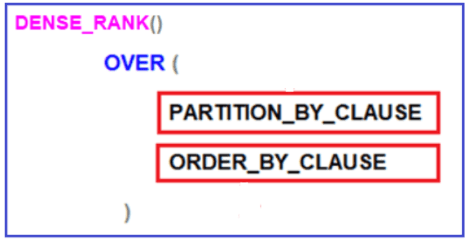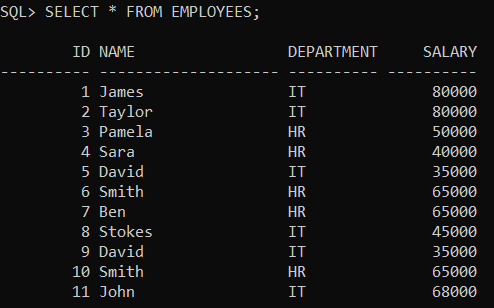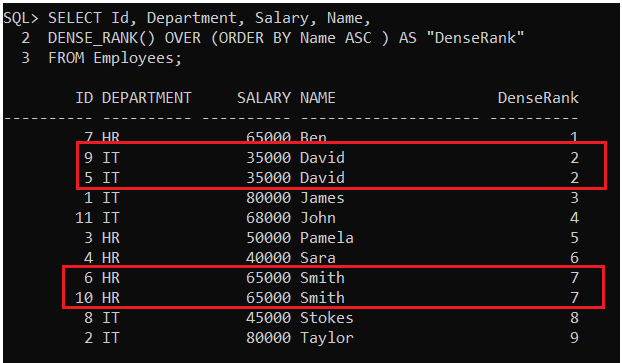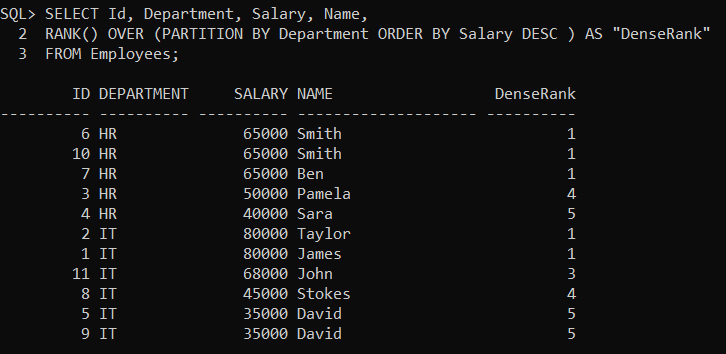# DENSE_RANK Function in Oracle

## DENSE_RANK Function in Oracle with Examples

In this article, I am going to discuss DENSE_RANK Function in Oracle with Examples. Please read our previous article where we discussed RANK Function in Oracle with Examples. At the end of this article, you will understand what exactly the Dense Rank function is and when and how to use the Dense Rank function in Oracle with real-time examples.

##### DENSE_RANK Function in Oracle

The DENSE_RANK Function in Oracle is used to return sequential numbers starting from 1 based on the ordering of rows imposed by the ORDER BY clause. When we have two records with the same data, then it will give the same rank to both the rows. The following is the syntax to use the DENSE RANK function in Oracle. As you can see, like the RANK and Row Number functions, here also the Partition by clause is optional and the Order by Clause is mandatory.The PARTITION BY clause is used to partition the result set into multiple Partitions. As it is optional, if we did not specify the PARTITION BY clause, then the DENSE_RANK function will treat the entire result set as a single partition or single group. The ORDER BY clause is mandatory and is used to define the sequence in which each row is going to assign their DENSE RANK i.e. number.

Note: The DENSE RANK function in Oracle returns an increasing unique number for each row starting from 1 and the same for each partition. When there are duplicates or ties, the same rank will be assigned to all the duplicate rows, but the next row after the duplicate or tie rows have been assigned with the immediate next rank. So, the DENSE_RANK function in Oracle will not skip ranking if there are duplicates or tie rows.

##### Examples to understand the Dense Rank function in Oracle:

We are going to use the following Employees table to understand the DENSE RANK function in Oracle with examples.Please use the following SQL Script to create and populate the Employees table with the required sample data.

```CREATE TABLE Employees
(
Id INT PRIMARY KEY,
Name VARCHAR2(20),
Department VARCHAR2(10),
Salary INT
);

Insert Into Employees Values (1, 'James', 'IT', 80000);
Insert Into Employees Values (2, 'Taylor', 'IT', 80000);
Insert Into Employees Values (3, 'Pamela', 'HR', 50000);
Insert Into Employees Values (4, 'Sara', 'HR', 40000);
Insert Into Employees Values (5, 'David', 'IT', 35000);
Insert Into Employees Values (6, 'Smith', 'HR', 65000);
Insert Into Employees Values (7, 'Ben', 'HR', 65000);
Insert Into Employees Values (8, 'Stokes', 'IT', 45000);
Insert Into Employees Values (9, 'David', 'IT', 35000);
Insert Into Employees Values (10, 'Smith', 'HR', 65000);
Insert Into Employees Values (11, 'John', 'IT', 68000);
```
##### DENSE_RANK Function without PARTITION BY Clause in Oracle

Let us first see an example of the DENSE_RANK function without using the PARTITION BY Clause in Oracle. If we did not specify the PARTITION BY Clause, then the DENSE_RANK function will treat the entire result set as a single partition and give consecutive numbering starting from 1 except when there is a tie. The following is an example of the DENSE_RANK function in Oracle without using the PARTITION BY clause. Here we use the Order by Clause on the Name column. So, it will give the rank based on the Name column.

```SELECT Id, Department, Salary, Name,
DENSE_RANK() OVER (ORDER BY Name ASC ) AS "DenseRank"
FROM Employees;
```

When you execute the above query, you will get the following output. As you can see in the below output, there will be no partition and hence all the rows are assigned with consecutive integer numbers starting from 1 except when there is a tie i.e. for the Employee name David, and Smith, it gives the same rank to both the rows.The Dense Rank function in Oracle will not skip the ranking when there is a tie. As you can see in the above output, we have two employees with the rank of 2 but the next employee Rank is 3, and again, we have two employees with the rank 7 but the next immediate rank is 8.

##### DENSE_RANK Function with PARTITION BY clause in Oracle:

Now, let us see an example of the DENSE_RANK function using the PARTITION BY clause in Oracle. When we specify the PARTITION BY Clause, then the result set is partitioned based on the column which we specify in the Partition BY clause. Please have a look at the following example for a better understanding. As you can see, we have specified Department in the Partition By clause and Salary in the Order By clause. As in our Employees table, we have two departments (IT and HR). So, the Partition by Clause will divide the result set into two partitions. One partition is for IT department employees and the other partition is for the HR department employees. Then on each partition, the data is sorted based on the Salary column. The Oracle DENSE_RANK function is then applied on each partition and gives an integer sequence number starting from 1 to each record except when there is a tie. In the case of a tie, it gives the same rank and then continues with the sequence without skipping any rank or number.

```SELECT Id, Department, Salary, Name,
RANK() OVER (PARTITION BY Department ORDER BY Salary DESC ) AS "DenseRank"
FROM Employees;
```

Now, when you execute the above code, you will get the following output.##### What is the difference between RANK and DENSE_RANK functions in Oracle?

As we already discussed the one and only difference between the RANK and DENSE_RANK functions in Oracle is that the RANK function skips ranking(s) if there is a tie whereas the DENSE_RANK function will not skip the ranking in oracle when there is a tie.

##### Real-time Examples of DENSE_RANK Functions in Oracle

If you are attending any interview, then one famous question is being asked in almost all interviews i.e. find the nth highest salary. The DENSE_RANK functions can be used to find the nth highest salary. Let us understand this with an example. Suppose, there are 2 employees with the FIRST highest salary, then there might be 2 business scenarios as follows:

1. If your business requirement is not to produce any result for the SECOND highest salary then you have to use the RANK function.
2. If your business requirement is to return the next salary after the tied rows as the SECOND highest Salary, then you have to use the DENSE_RANK function.
##### Fetch the 2nd Highest Salary using the DENSE RANK function in Oracle:

As we have 2 Employees with the FIRST highest salary i.e. 80000, the DENSE_RANK() function will return the next Salary after the tied rows as the SECOND highest Salary i.e. 68000. Please execute the following SQL Script and see the output.

```WITH EmployeeCTE  AS
(
SELECT Salary,
DENSE_RANK() OVER (ORDER BY Salary DESC) AS Rank_Salry
FROM Employees
)
SELECT Salary FROM EmployeeCTE WHERE Rank_Salry = 2 FETCH FIRST 1 ROWS ONLY;
```

Output: 68000

##### Example to find the nth Highest Salary Department wise:

You can also use the DENSE_RANK functions in Oracle to find the nth highest Salary department-wise. For example, if someone asks you to find the 3rd highest salary of the HR Department, then you can use the DENSE_RANK function as shown below.

```WITH EmployeeCTE  AS
(
SELECT Salary, Department,
DENSE_RANK() OVER (PARTITION BY Department ORDER BY Salary DESC)
AS Salary_Rank
FROM Employees
)
SELECT Salary FROM EmployeeCTE WHERE Salary_Rank = 3 AND Department = 'HR' FETCH FIRST 1 ROWS ONLY;
```

Output: 40000

So, in short, the DENSE_RANK function in Oracle is used to return an increasing sequential integer number for each row starting from 1 and the same for each partition. When there are duplicates, then the same rank is assigned to all the duplicate rows but it will not skip any ranks. This means the next row after the duplicate rows will have the next rank in the sequence.

In the next article, I am going to discuss ANY Operator in Oracle with Examples. Here, in this article, I try to explain the need and use of the DENSE_RANK Function in Oracle with Examples. I hope you enjoy this Oracle DENSE_RANK Function article.